# Java Program to Count Duplicate Elements in Array

In this tutorial you will learn about the Java Program to Count Duplicate Elements in Array and its application with practical example.

## Java Program to Count Duplicate Elements in Array

In this tutorial, we will learn to create a Java program that will count the duplicate elements of an array using Java programming.

## Prerequisites

Before starting with this tutorial, we assume that you are the best aware of the following Java programming topics:

• Operators in Java Programming.
• Basic Input and Output function in Java Programming.
• Basic Java programming.
• For loop in Java programming.
• Arithmetic operations in Java Programming.

## Printing the duplicate Elements in an Array.

In this program, First, we will first take the size of the array from the user. Then we will take the elements of the array from the user. After that, we will use the conditional statements and the loops to check the duplicate elements of the array. Then we will be using a for loop to print the duplicate elements of the array.

With the help of this program, we can be print the duplicate elements of the array.

## Output:-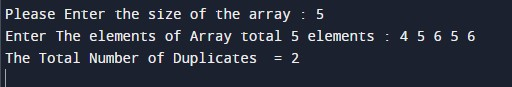In the above program, we have first initialized the required variable.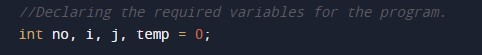• no = it will hold the integer value.
• i = it will hold the integer value.
• j = it will hold the integer value.
• temp = it will hold the integer value.

Taking the size of the array from the user.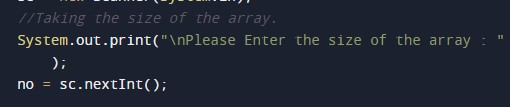Taking the input elements for the array.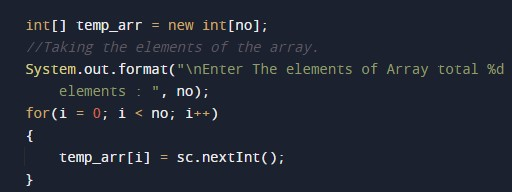Finding the duplicate number of elements of the array.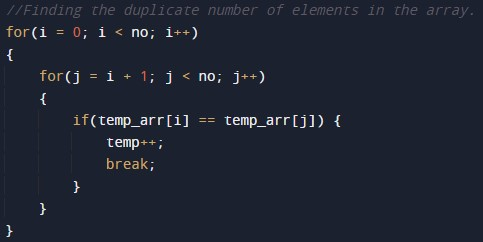Printing output duplicate number of elements of the array.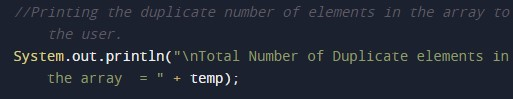In this tutorial we have learn about the Java Program to Count Duplicate Elements in Array and its application with practical example. I hope you will like this tutorial.